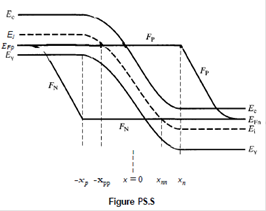### Create an Account

Home / Questions / The energy band diagram for a reverse biased Si pn junction diode under steady state condi...

# The energy band diagram for a reverse biased Si pn junction diode under steady state conditions is pictured in

The energy band diagram for a reverse-biased Si pn-junction diode under steady-state conditions is pictured in Fig. P5.5.(a) With the aid of the diagram and assuming single-level R-G center statistics, Tn = TP = T, and ET" = Ei, simplify the general steady-state net recombination rate expression to obtain the simplest possible relationship for R at (i) x = 0, (ii) x = - xp,

(iii) x = x nn " (iv) x = - x p, and (v) x = xn•

(b) Sketch R versus x for x-values lying within the electrostatic depletion region (- xp ≤ X ≤ xn).

(c) What was the purpose or point of this problem?

Jul 25 2020 View more View LessSubscribe To Get Solution